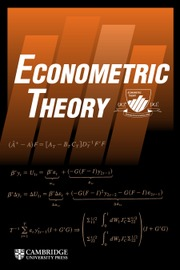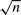Home
Hostname: page-component-684899dbb8-ndjvl Total loading time: 0.326 Render date: 2022-05-26T02:43:12.304Z Has data issue: true Feature Flags: { "shouldUseShareProductTool": true, "shouldUseHypothesis": true, "isUnsiloEnabled": true, "useRatesEcommerce": false, "useNewApi": true }Econometric Theory

# AVERAGING OF AN INCREASING NUMBER OF MOMENT CONDITION ESTIMATORS

Published online by Cambridge University Press:  29 December 2014

*
*Address correspondence to Oliver Linton, Department of Economics, University of Cambridge, Austin Robinson Building, Sidgwick Avenue, Cambridge CB3 9DD, United Kingdom; e-mail: obl20@cam.ac.uk.

## Abstract

HTML view is not available for this content. However, as you have access to this content, a full PDF is available via the ‘Save PDF’ action button.

We establish the consistency and asymptotic normality for a class of estimators that are linear combinations of a set of$\sqrt n$-consistent nonlinear estimators whose cardinality increases with sample size. The method can be compared with the usual approaches of combining the moment conditions (GMM) and combining the instruments (IV), and achieves similar objectives of aggregating the available information. One advantage of aggregating the estimators rather than the moment conditions is that it yields robustness to certain types of parameter heterogeneity in the sense that it delivers consistent estimates of the mean effect in that case. We discuss the question of optimal weighting of the estimators.

Type
ARTICLES
Information
Econometric Theory , February 2016 , pp. 30 - 70

## References

Antoine, B. & Renault, E. (2012) Efficient minimum distance estimation with multiple rates of convergence. Journal of Econometrics 170(2), 350367.CrossRefGoogle Scholar
Belloni, A., Chen, D., Chernozhukov, V., & Hansen, C. (2012) Spare models and methods for optimal instruments with an application to eminent domain. Econometrica 80(6), 23692429.Google Scholar
Bickel, P.J. & Levina, E. (2008) Covariance regularization by thresholding. Annals of Statistics 36(6), 25772604.CrossRefGoogle Scholar
Bierens, H.J. (1987) Kernel estimators of regression functions. In Bewley, T.F. (ed.), Advances in Econometrics – Fifth World Congress of the Econometric Society, vol. I of Econometric Society Monographs, 1st ed., chap. 3, pp. 99144. Cambridge University Press.Google Scholar
Breiman, L. (1996) Bagging predictors. Machine Learning 24(2), 123140.CrossRefGoogle Scholar
Breiman, L. (1999) Using Adaptive Bagging to Debias Regressions. Technical report 547, Department of Statistics, University of California Berkeley.
Cai, T.T., Liu, W., & Zhou, H.H. (forthcoming) Estimating sparse precision matrix: Optimal rates of convergence and adaptive estimation. Annals of Statistics.Google Scholar
Campbell, J.Y., Lo, A., & Mackinlay, A.C. (1997) The Econometrics of Financial Markets, 1st ed. Princeton University Press.Google Scholar
Chamberlain, G. (1987) Asymptotic efficiency in estimation with conditional moment restrictions. Journal of Econometrics 34, 305334.CrossRefGoogle Scholar
Chamberlain, G. (1992) Efficiency bounds for semiparametric regression. Econometrica 60(3), 567596.CrossRefGoogle Scholar
Chen, X., Jacho-Chávez, D.T., & Linton, O.B. (2009) An Alternative Way of Computing Efficient Instrumental Variable Estimators. Sticerd Working paper EM/2009/536, STICERD.
Chen, X. & Pouzo, D. (2009) Efficient estimation of semiparametric conditional moment models with possibly nonsmooth residuals. Journal of Econometrics 152(1), 4660.CrossRefGoogle Scholar
Chesher, A. (1984) Testing for neglected heterogeneity. Econometrica 52(4), 865872.CrossRefGoogle Scholar
Donald, S.G., Imbens, G.W., & Newey, W.K. (2003) Empirical likelihood estimation and consistent tests with conditional moment restrictions. Journal of Econometrics 117(1), 5593.CrossRefGoogle Scholar
Donald, S.G. & Newey, W.K. (2001) Choosing the number of instruments. Econometrica 69(5), 11611191.CrossRefGoogle Scholar
Fan, J., Feng, Y., & Song, R. (2011) Nonparametric independence screening in sparse ultra-high-dimensional additive models. Journal of the American Statistical Association 106(494), 544557.CrossRefGoogle ScholarPubMed
Fan, J., Li, Y., & Yu, K. (2012) Vast volatility matrix estimation using high-frequency data for portfolio selection. Journal of the American Statistical Association 107(497), 412428.CrossRefGoogle ScholarPubMed
Fan, J. & Lv, J. (2008) Sure independence screening for ultrahigh dimensional feature space. Journal of the Royal Statistical Society, Series B 70, 849911.CrossRefGoogle Scholar
Granger, C.W.J. & Jeon, Y. (2004) Thick modeling. Economic Modelling 21(2), 323343.CrossRefGoogle Scholar
Gray, H.L. & Schucany, W.R. (1972) The Generalized Jackknife Statistic, 1st ed. Marcel Dekker.Google Scholar
Han, C. & Phillips, P.C.B. (2006) GMM with many moment conditions. Econometrica 74(1), 147192.CrossRefGoogle Scholar
Hansen, L.P. (1982) Large sample properties of generalized methods of moments estimators. Econometrica 50, 10291054.CrossRefGoogle Scholar
Hansen, L.P. (1985) A method for calculating bounds on the asymptotic covariance matrices of generalized method of moments estimators. Journal of Econometrics 30(1–2), 203238.CrossRefGoogle Scholar
Hansen, B.E. (2007) Least squares model averaging. Econometrica 75(4), 11751189.CrossRefGoogle Scholar
Hansen, B.E. (2008) Least-squares forecast averaging. Journal of Econometrics 146(2), 342350.CrossRefGoogle Scholar
Hansen, B.E. (2009) Averaging estimators for regressions with a possible structural break. Econometric Theory 25(06), 14981514.CrossRefGoogle Scholar
Hansen, B.E. (2010) Averaging estimators for autoregressions with a near unit root. Journal of Econometrics 158(1), 142155.CrossRefGoogle Scholar
Hansen, B.E. & Racine, J.S. (2012) Jackknife model averaging. Journal of Econometrics 167(1), 3846.CrossRefGoogle Scholar
Härdle, W. & Stoker, T.M. (1989) Investigating smooth multiple regression by the method of average derivatives. Journal of the American Statistical Association 84(408), 986995.Google Scholar
Huber, P.J. (1967) The behavior of maximum likelihood estimates under nonstandard conditions. In Cam, L.M.L. & Neyman, J. (eds.), Proceedings of the Fifth Berkeley Symposium on Mathematical Statistics and Probability, Volume 1: Statistics, pp. 221233. Statistical Laboratory of the University of California, Berkeley. University of California Press.Google Scholar
Koenker, R.W. & Bassett, G.. (1978) Regression quantiles. Econometrica 46(1), 3350.CrossRefGoogle Scholar
Koenker, R. & Machado, J.A.F. (1999) GMM inference when the number of moment conditions is large. Journal of Econometrics 93(2), 327344.CrossRefGoogle Scholar
Kotlyarova, Y. & Zinde-Walsh, V. (2006) Non- and semi-parametric estimation in models with unknown smoothness. Economics Letters 93(3), 379386.CrossRefGoogle Scholar
Kotlyarova, Y. & Zinde-Walsh, V. (2007) Robust kernel estimator for densities of unknown smoothness. Journal of Nonparametric Statistics 19(2), 89101.CrossRefGoogle Scholar
Koul, H.L. & Stute, W. (1999) Nonparametric model checks for time series. Annals of Statistics 27(1), 204236.Google Scholar
Kuersteiner, G. & Okui, R. (2010) Constructing optimal instruments by first-stage prediction averaging. Econometrica 78(2), 697718.Google Scholar
Ledoit, O. & Wolf, M. (2004) A well-conditioned estimator for large dimensional covariance matrices. Journal of Multivariate Analysis 88(2), 365411.CrossRefGoogle Scholar
Lee, L. (2010) Pooling estimates with different rates of convergence – the minimum χ2 approach with an emphasis on a social interactions model. Econometric Theory 26, 260299.CrossRefGoogle Scholar
Lee, Y. & Zhou, Y. (2011) Averaged Instrumental Variables Estimator. Unpublished manuscript.
Linton, O.B. & Nielsen, J.P. (1995) A kernel model of estimating structured nonparametric regression based on marginal integration. Biometrika 82, 93100.CrossRefGoogle Scholar
Lobato, I.N. & Dominguez, M.A. (2004) Consistent estimation of models defined by conditional moment restrictions. Econometrica 72(5), 16011615.Google Scholar
Malinvaud, E. (1966) Statistical Methods of Econometrics, 1st ed. Studies in Mathematical and Managerial Economics. Rand McNally.Google Scholar
McFadden, D. (1989) A method of simulated moments for estimation of discrete response models without numerical integration. Econometrica 57(5), 9951026.CrossRefGoogle Scholar
Newey, W.K. (1990) Efficient instrumental variables estimation of nonlinear models. Econometrica 58(4), 809837.CrossRefGoogle Scholar
Newey, W.K. (1993) Efficient estimation of models with conditional moment restrictions. In Maddala, G.S., Rao, C.R., & Vinod, H.D. (eds.), Handbook of Statistics, 1st ed., vol. 11, pp. 419454. Elsevier.Google Scholar
Newey, W.K. & McFadden, D. (1994) Large sample estimation and hypothesis testing. In McFadden, D. & Engle, R.F. (eds.), Handbook of Econometrics, vol. IV, pp. 21112245. Elsevier.Google Scholar
Newey, W.K. & Smith, R.J. (2004) Higher order properties of GMM and generalized empirical likelihood estimators. Econometrica 72(1), 219255.CrossRefGoogle Scholar
Okui, R. (2011) Instrumental variable estimation in the presence of many moment conditions. Journal of Econometrics 165, 7086.CrossRefGoogle Scholar
Pakes, A. & Pollard, D. (1989) Simulation and the asymptotics of optimization estimators. Econometrica 57(5), 10271057.CrossRefGoogle Scholar
Pesaran, M.H. (2006) Estimation and inference in large heterogeneous panels with a multifactor error structure. Econometrica 74(4), 9671012.CrossRefGoogle Scholar
Phillips, P.C.B. (1983) Exact small sample theory in the simultaneous equations model. In Griliches, Z. & Intriligator, M.D. (eds.), Handbook of Econometrics, 1st ed., vol. 1, chap. 8, pp. 449516. Elsevier.Google Scholar
Phillips, P.C.B. & Moon, H.R. (1999) Linear regression limit theory for nonstationary panel data. Econometrica 67(5), 10571112.CrossRefGoogle Scholar
Politis, D.N. & Romano, J.P. (1992) A general resampling scheme for triangular arrays of α-mixing random variables with application to the problem of spectral density estimation. Annals of Statistics 20(4), 19852007.CrossRefGoogle Scholar
Portnoy, S. (1984) Asymptotic behavior of M-estimators of p regression parameters when p2/n is large. I. Consistency. Annals of Statistics 12(4), 12981309.CrossRefGoogle Scholar
Rilstone, P., Srivastava, V.K., & Ullah, A. (1996) The second-order bias and mean squared error of nonlinear estimators. Journal of Econometrics 75(2), 369395.CrossRefGoogle Scholar
Rilstone, P. & Ullah, A. (2005) Corrigendum to ‘The second-order bias and mean squared error of nonlinear estimators’; [Journal of Econometrics 75(2) (1996) 369–395]. Journal of Econometrics 124(1), 203204.CrossRefGoogle Scholar
Rothenberg, T.J. (1973) Efficient Estimation with A Priori Information, 1st ed. Yale University Press.Google Scholar
Rothenberg, T.J. (1974) A Note on Two-Stage Least Squares. Unpublished manuscript.
Sawa, T. (1973) Almost unbiased estimator in simultaneous equations systems. International Economic Review 14(1), 97106.CrossRefGoogle Scholar
Schafgans, M.M.A. & Zinde-Walsh, V. (2010) Smoothness adaptive average derivative estimation. Econometrics Journal 13(1), 4062.CrossRefGoogle Scholar
Shorack, G.R. & Wellner, J.A. (2009) Empirical Processes with Applications to Statistics, 1st ed. Classics in Applied Mathematics. Society for Industrial & Applied Mathematics.
Stock, J.H. & Watson, M.W. (1999) Forecasting inflation. Journal of Monetary Economics 44(2), 293335.CrossRefGoogle Scholar
Stock, J.H. & Wright, J. (2000) GMM with weak identification. Econometrica 68(5), 10551096.CrossRefGoogle Scholar
Swamy, P.A.V.B. (1970) Efficient inference in a random coefficient regression model. Econometrica 38(2), 311323.CrossRefGoogle Scholar
Watson, M.W. (2003) Macroeconomic forecasting using many predictors. In Dewatripont, M., Hansen, L.P., & Turnovsky, S.J. (eds.), Advances in Economics and Econometrics: Theory and Applications – Eighth World Congress of the Econometric Society, vol. III of Econometric Society Monographs, 1st ed., chap. 3, pp. 87115. Cambridge University Press.CrossRefGoogle Scholar
Zhang, L., Mykland, P.A., & Ait-Sahalia, Y. (2005) A tale of two time scales: Determining integrated volatility with noisy high-frequency data. Journal of the American Statistical Association 100, 13941411.CrossRefGoogle Scholar
Zhao, Z. & Xiao, Z. (2014) Efficient regression via optimally combining quantile information. Econometric Theory 30(6), 12721314.CrossRefGoogle ScholarPubMedYou have Access
10
Cited by

# Save article to Kindle

Note you can select to save to either the @free.kindle.com or @kindle.com variations. ‘@free.kindle.com’ emails are free but can only be saved to your device when it is connected to wi-fi. ‘@kindle.com’ emails can be delivered even when you are not connected to wi-fi, but note that service fees apply.

Find out more about the Kindle Personal Document Service.

AVERAGING OF AN INCREASING NUMBER OF MOMENT CONDITION ESTIMATORS
Available formats
×

# Save article to Dropbox

To save this article to your Dropbox account, please select one or more formats and confirm that you agree to abide by our usage policies. If this is the first time you used this feature, you will be asked to authorise Cambridge Core to connect with your Dropbox account. Find out more about saving content to Dropbox.

AVERAGING OF AN INCREASING NUMBER OF MOMENT CONDITION ESTIMATORS
Available formats
×

# Save article to Google Drive

To save this article to your Google Drive account, please select one or more formats and confirm that you agree to abide by our usage policies. If this is the first time you used this feature, you will be asked to authorise Cambridge Core to connect with your Google Drive account. Find out more about saving content to Google Drive.

AVERAGING OF AN INCREASING NUMBER OF MOMENT CONDITION ESTIMATORS
Available formats
×
×